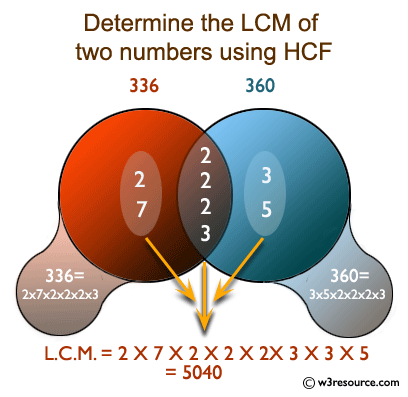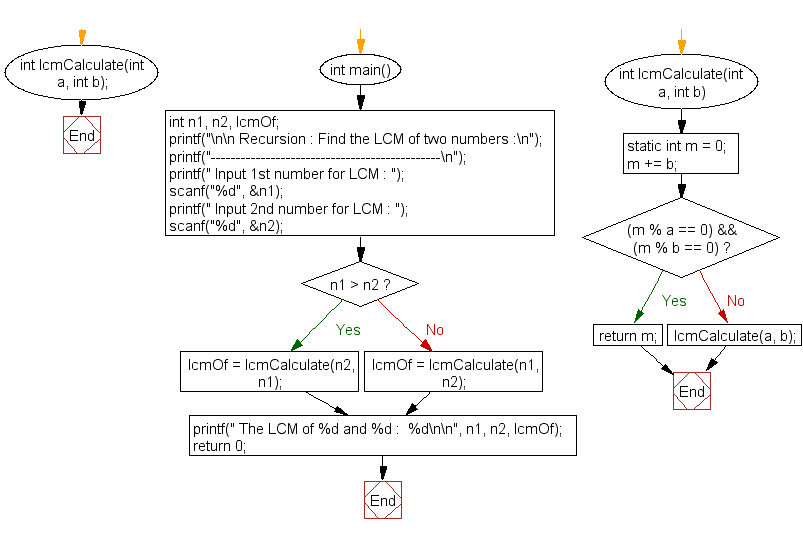﻿ C Program: Find the LCM of two numbers - w3resource

# C Exercises: Find the LCM of two numbers

## C Recursion: Exercise-13 with Solution

Write a program in C to find the LCM of two numbers using recursion.

Pictorial Presentation:Sample Solution:

C Code:

``````#include <stdio.h>
int lcmCalculate(int a, int b);

int main()
{
int n1, n2, lcmOf;
printf("\n\n Recursion : Find the LCM of two numbers :\n");
printf("----------------------------------------------\n");
printf(" Input 1st number for LCM : ");
scanf("%d", &n1);
printf(" Input 2nd number for LCM : ");
scanf("%d", &n2);
// Ensures that first parameter of lcm must be smaller than 2nd
if(n1 >  n2)
lcmOf = lcmCalculate(n2, n1);//call the function lcmCalculate for lcm calculation
else
lcmOf = lcmCalculate(n1, n2);//call the function lcmCalculate for lcm calculation
printf(" The LCM of %d and %d :  %d\n\n", n1, n2, lcmOf);
return 0;
}
int lcmCalculate(int a, int b)//the value of n1 and n2 is passing through a and b
{
static int m = 0;
//Increments m by adding max value to it
m += b;
//  If found a common multiple then return the m.
if((m % a == 0) && (m % b == 0))
{
return m;
}
else
{
lcmCalculate(a, b);//calling the function lcmCalculate itself
}
}
```
```

Sample Output:

``` Recursion : Find the LCM of two numbers :
----------------------------------------------
Input 1st number for LCM : 4
Input 2nd number for LCM : 6
The LCM of 4 and 6 :  12
```

Explanation:

```int lcmCalculate(int a, int b)//the value of n1 and n2 is passing through a and b
{
static int m = 0;
//Increments m by adding max value to it
m += b;
//  If found a common multiple then return the m.
if((m % a == 0) && (m % b == 0))
{
return m;
}
else
{
lcmCalculate(a, b);//calling the function lcmCalculate itself
}
}
```

This function lcmCalculate() calculates the least common multiple (LCM) of two numbers. It takes two integers ‘a’ and ‘b’ as input and calculates the LCM of these two numbers using recursion.

The function starts by initializing a static variable m to zero. It then increments m by adding the value of b to it. This is done repeatedly until a common multiple of a and b is found.

Once a common multiple is found, the function returns the value of m as the LCM. If a common multiple is not found, the function calls itself recursively to continue the search for a common multiple.

Time complexity and space complexity:

The time complexity of this function is O(a*b), where a and b are the two input numbers.

The space complexity is O(1), as the function uses a static variable to store the value of m.

Flowchart:C Programming Code Editor:

Have another way to solve this solution? Contribute your code (and comments) through Disqus.

﻿

## C Programming: Tips of the Day

__FILE__ macro shows full path

```#include <string.h>

#define __FILENAME__ (strrchr(__FILE__, '/') ? strrchr(__FILE__, '/') + 1 : __FILE__)
For Windows use '\\' instead of '/'.
```

Ref : https://bit.ly/3iEWRoT

We are closing our Disqus commenting system for some maintenanace issues. You may write to us at reach[at]yahoo[dot]com or visit us at Facebook

Test your Programming skills with w3resource's quiz.

﻿

## C Programming: Tips of the Day

__FILE__ macro shows full path

```#include <string.h>

#define __FILENAME__ (strrchr(__FILE__, '/') ? strrchr(__FILE__, '/') + 1 : __FILE__)
For Windows use '\\' instead of '/'.
```

Ref : https://bit.ly/3iEWRoT

We are closing our Disqus commenting system for some maintenanace issues. You may write to us at reach[at]yahoo[dot]com or visit us at Facebook